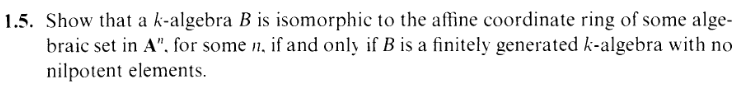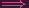I.1.5

12/4/2020What a fucking word salad, but it's easy. It took me like 5 minutes. Regardless, that's all I'm doing for today, even though I'm behind. Yes, yes, I'm a lazy slowpoke. Yes, yes, I always go too easy on myself. I self-deprecate a lot, but if I had a clone, I'd pamper it.

So we have here a characterization of affine coordinate rings, eh? I've heard that said coordinate rings should be thought of as "functions" (kn k) on the corresponding zero set, so maybe I can sometimes think of the coordinate ring as a "function space" or "function ring". In which case, I suppose the sense of this exercise advances slightly past a merely dry algebra computation. For any An, we know that, on any zero set in it, the ring of kn k (polynomial) functions is just an *AHEM* "finitely generated k-algebra with no nilpotents"... Ouch, I guess it degenerated into word salad again, but I guess words can only express so much *puckers lips*. No? Okay *unpuckers lips* I've also heard that schemes and modern algebraic geometry "matter" partly because they can "capture" nilpotents, and I guess what we're dealing with here is the nice, classical case where we don't have nilpotents... or something. I'll keep an eye on those nilpotents, is all I'm saying.

Anyway, here's the proof.

It's easy to check that
(1) B = A∕J has no nilpotents ⇐⇒ the J is radical.
(so, from strongest to weakest, maximal ⇐⇒ field, prime ⇐⇒ integral domain, radical ⇐⇒ no nilpotents)

So... to show thedirection,
we have

B = A(Y ) where Y is some algebraic set in some An so A(Y ) = k[x1,...,xn]∕I(Y ) where I(Y ) is a radical ideal, but this means that B is a finitely generated k-alg with no nilpotents (by (1))

Sois done.

For the other direction, we are given,
B = k[x1,...,xn]∕J where J is radical (by (1)). Let Y = Z(J) in An so that

 A(Y ) = k[x1,...,xn]∕I(Y ) = k[x1,...,xn]∕I(Z(J)) = k[x1,...,xn]∕J = B

Soo = is done.

Also, yes, I'm late to getting around to this one, as usual. Guess I'm always late to the party, heh *puts on sunglasses* and I know in 1.1b I said I would only do 5 per section, but I looked ahead and it looks like some of the other exercises in this section are actually kinda important, so I guess I'm doing most of them after all *shamefully removes sunglasses*.

ERRATA on 1.4:
In 1.4, I said that Z(y2 - x2 - 1) is the unit circle. Shoulda been Z(y2 + x2 - 1), lol